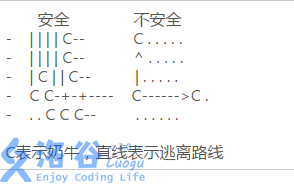# 题目

## 题目描述## 输入输出格式

### 输入格式

$1$行：两个整数$r,c$，用1个空格隔开，表示矩形的行数和列数（均 $\le 50$）。
$2$行：一个整数$n$，表示奶牛的个数（$\le 100$）。
$3$$n+2$行：共$n$行，每行有$2$个整数，之间用$1$个空格隔开，分别表示这头奶牛所在的行和列。

5 5
5
1 1
2 4
3 1
2 2
2 1

1
5

# 题解

#include<iostream>//有漏洞
#include<cstring>
#include<cstdio>
using namespace std;
int m,h,l,n;
struct cow{     //结构体，也可以用x和y
int x,y;
};
cow a;
int anquan(int x,int y){     //每头奶牛安全的条件
int t1=1,t2=1;
for(int i=1;i<x;i++) if(m[i][y]) {t1=0;break;}     //如果北面有奶牛，那么t1=0
for(int i=y+1;i<=l;i++) if(m[x][i]) {t2=0;break;}     //如果东面有奶牛，那么t2=0
if(t1==0&&t2==0) return 0; else return 1;     //如果两边都有奶牛，则不安全，反之安全
}
int qaq(){     //所有奶牛都安全
for(int i=1;i<=h;i++) for(int j=1;j<=l;j++) if(m[i][j]) if(!(anquan(i,j))) return 0;     //遍历整个农场，如果有奶牛不安全，那么返回0
return 1;     //否则返回1
}
int aq(int x,int y){     //去掉一只奶牛后安全
m[x][y]=0;     //删除奶牛
return qaq();
}
int main()
{
int temp=0;
char s;
cin>>h>>l>>n;
memset(a,0,sizeof(a));memset(m,0,sizeof(m));     //初始化
for(int i=1;i<=n;i++){
cin>>a[i].x>>a[i].y;     //输入
m[a[i].x][a[i].y]=i;     //也可以赋值为1
gets(s);
}
if(qaq()) {cout<<0;return 0;}     //如果全部安全，输出0，结束
for(int i=1;i<=n;i++){
if(aq(a[i].x,a[i].y)) /*如果去掉后安全*/if(!temp) /*如果是第一次输出*/cout<<i,temp++; else cout<<endl<<i;
m[a[i].x][a[i].y]=i;     //这步非常重要，不然第二次执行的时候就删除了两只奶牛
}
if(!temp) cout<<1;     //如果删掉任何一只奶牛都不安全，输出-1
return 0;
}


©️2019 CSDN 皮肤主题: 大白 设计师: CSDN官方博客